Sie sind hier: ICP » R. Hilfer » Publikationen

# 3 Definition of the Model

[681.1.8.1] In this section, a brief summary of the mathematical model is given. [681.1.8.2] The following equations are based on volume, mass and momentum balance equations analogous to the foundations of the traditional theory (see e.g.  for a succinct but detailed parallel development). [681.1.8.3] It is assumed that both fluids are incompressible and immiscible and that the lateral dimensions of the column are small with respect to the capillary fringe. [681.1.8.4] Hence, a one dimensional description is an appropriate approximation. [681.1.8.5] The mass balances for the four fluid phases (percolating water is identified by the index 1, non-percolating water by 2, percolating oil by 3 and non-percolating oil by 4) read as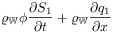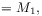(1a)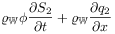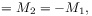(1b)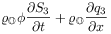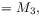(1c)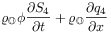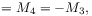(1d)

where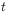denotes time,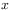denotes position or height along the column,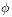denotes porosity,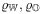denote the density of water, respectively air,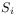the saturation,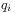the volume flux and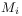the mass exchange term of the phase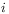. [681.1.8.6] The mass exchange term accounts for the fact that percolating and non-percolating phases of the same fluid exchange mass by break-up and coalescence. [681.1.8.7] The mass exchange terms take the form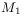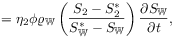(2a)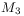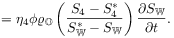(2b)

with the parameter functions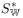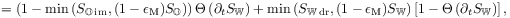(3a)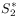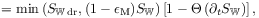(3b)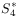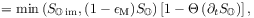(3c)

where the parameter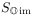is a limiting saturation for the non-percolating air,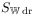a limiting saturation for non-percolating water and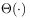denotes the Heaviside step function. [681.1.8.8] Water saturation is given by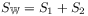and the air saturation by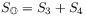. [681.1.8.9] The parameter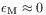is a mathematical regularization parameter. [681.1.8.10] It allows to simulate also primary processes. [681.1.8.11] The volume fluxes of the phases take the form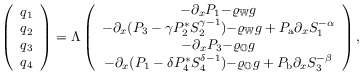(4)

where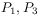denote the averaged pressures of the percolating phases,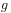the gravity acceleration,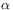,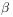,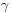,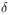,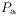,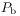,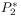,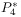, are constitutive parameters. [681.1.8.12] The parameters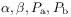are associated with capillary potentials and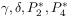with the energy stored in the interface between the non-percolating phases and the surrounding percolating phases of the other fluid . [681.1.8.13] A generalized mobility matrix is denoted by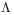with the coefficients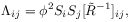(5)

where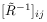denote the components of the inverse of the viscous coupling parameter matrix. [681.1.8.14] A comparison with the classical two-phase Darcy equations yields that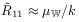and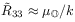, where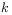denotes the permeability of the porous medium. [681.1.8.15] The system of equations is closed with the volume conservation for incompressible fluids and incompressible porous media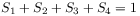(6)

plus a special form of the general self-consistent closure condition [11, 5]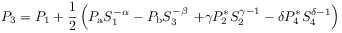(7)

for the pressures of the percolating phases.